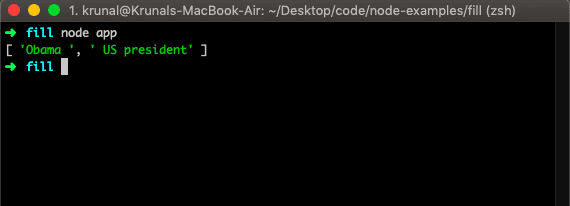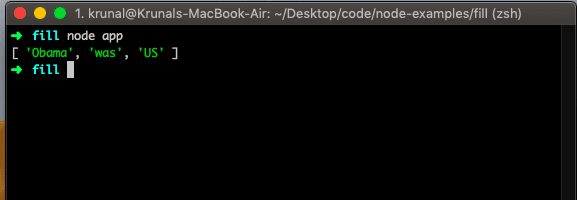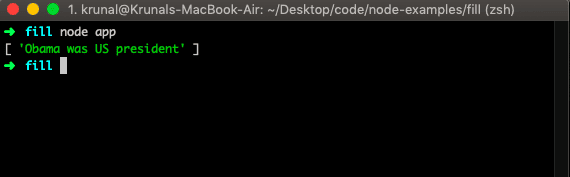# JavaScript split string: The Complete Guide

Javascript string split() method returns a new array. At each match of the separator .split() method will split the string and add the split section as an element to an array.

## JavaScript split string

To split a string in JavaScript, use the string split() function. The string split() is a built-in JavaScript function that splits the String object into an array of strings by separating a string into substrings, using a specified separator string to determine where to make each split.

The string split() function is used to split the given string into an array of strings by separating it into substrings using a specified separator provided in the argument.

If an empty string (”) is used as the separator, the string is split between each character. The string split() method does not change an original string.

### Syntax

The syntax for the split() method is the following.

`string.split(separator, limit)`

### Parameters

The separator is an optional parameter. The separator specifies a character, or a regular expression, to use for splitting a string. If omitted, then the entire string will be returned.

The limit is an optional parameter. It is an integer that specifies the number of splits, items after the split limit will not be included in the array.

Let us take a simple example of a string split in javascript.

```// app.js

let str = 'Obama was US president';
let result  = str.split('was');
console.log(result);```

So, in the above example, we have split the string from a was the separator. So the output looks like this.When found, the separator is removed from the string, and the substrings are returned in an array. If the separator is not found or is omitted, the array contains one element consisting of the entire string. If the separator is an empty string, a string is converted to an array of characters.

If the separator appears at the beginning or end of a string, or both, then the array begins, ends, or both begins and ends, respectively, with the empty string. Thus, if a string consists solely of one instance of the separator, the array consists of two empty strings. If the separator is an array, then Array is coerced to String and used as a separator.

## Returning a limited number of splits

Look at the following example.

```// app.js

let str = 'Obama was US president';
let result  = str.split(' ', 3);
console.log(result);```In the above example, we have set the limiter 3. So it will split and returns three elements of an array.

## Split string with a regular expression in JavaScript

To split a string with regular expression in JavaScript, use the string.split() function and pass the regular expression as an argument.

```// app.js

let str = 'Obama was US president';
let result  = str.split(/(\d)/);
console.log(result);```## Javascript string split by comma

You can use the JavaScript string split() method to split a string using a specific separator such as comma (,), space, etc. If the separator is an empty string, the string is converted to an array of characters.

See the following code example.

```// app.js

let st3 = 'Eleven,Mike,Hopper,Dustin,Will,Lucas';
let arr = st3.split(',');
console.log(arr);
```

See the following output.

```➜  es git:(master) ✗ node app
[ 'Eleven', 'Mike', 'Hopper', 'Dustin', 'Will', 'Lucas' ]
➜  es git:(master) ✗
```

So, in the above code, all the comma-separated strings become the array of elements.

## Split string in two on a given index

If you have a string that needs to split on the given index and then return both parts, separated by a comma. See the following code example.

```// app.js

const splitData = (value, index) => {
return value.substring(0, index) + "," + value.substring(index);
}

console.log(splitData('110470116021', 6));
```

Now, see the following output.

```➜  es git:(master) ✗ node app
110470,116021
➜  es git:(master) ✗
```

In the above function, we have passed the two parameters the value and index.

We have used the Javascript substring() function and using that function, we have divided the two strings at the given index and then concat those strings and return the string.

## Split a string with multiple separators in Javascript

To split a string with multiple separators in JavaScript, use the string.split() function with regular expression.

```// app.js

string = 'Millie Bobby Brown';
op = string.split(/[\s,]+/)
console.log(op);
```

See the following output.

```➜  es git:(master) ✗ node app
[ 'Millie', 'Bobby', 'Brown' ]
➜  es git:(master) ✗
```

So, the JavaScript split() method splits the string object into an array of strings by breaking up a string into the substrings.

1. The split() method is used to split a string into an array of substrings.
2. The split() method returns the new array.
3. The split() method doesn’t change the original string.

## JavaScript split by slash using regular expression

We can split string by slash by applying a regular expression.

See the following code example.

```// app.js

let mString = 'millie/bobby/brown';
let splits = mString.split(/[\\\/]/);
console.log(splits);
```

See the following output.

```➜  es git:(master) ✗ node app
[ 'millie', 'bobby', 'brown' ]
➜  es git:(master) ✗
```

## JavaScript String Split at uppercase letters

Now, we will take a scenario in which we will split the string at the occurrence of the Uppercase characters. See the below code.

```// app.js

let mString = 'MillieBobbyBrown';
let splits = mString.split(/(?=[A-Z])/);
console.log(splits);
```

See the following output.

```➜  es git:(master) ✗ node app
[ 'Millie', 'Bobby', 'Brown' ]
➜  es git:(master) ✗
```

## JavaScript split to get the first element

We can split the string into an array of elements and get the first element using the following code.

```// app.js

let mString = 'MillieBobbyBrown';
let splits = mString.split(/(?=[A-Z])/);
console.log(splits);
```

See the following output.

```➜  es git:(master) ✗ node app
Millie
➜  es git:(master) ✗
```

## Javascript split a string into chunks

Let’s take an example in which we split the string into small chunks.

Here, we will not use the split() function but instead, we will use the Javascript String match() function.

See the following code.

```// app.js

let mString = 'MillieBobbyBrown';
let splits = mString.match(/.{1,2}/g);
console.log(splits);
```

See output.

```➜  es git:(master) ✗ node app
[ 'Mi', 'll', 'ie', 'Bo', 'bb', 'yB', 'ro', 'wn' ]
➜  es git:(master) ✗
```

The match() function retrieves the result of matching the string against the regular expression.

That’s it for this tutorial.

This site uses Akismet to reduce spam. Learn how your comment data is processed.